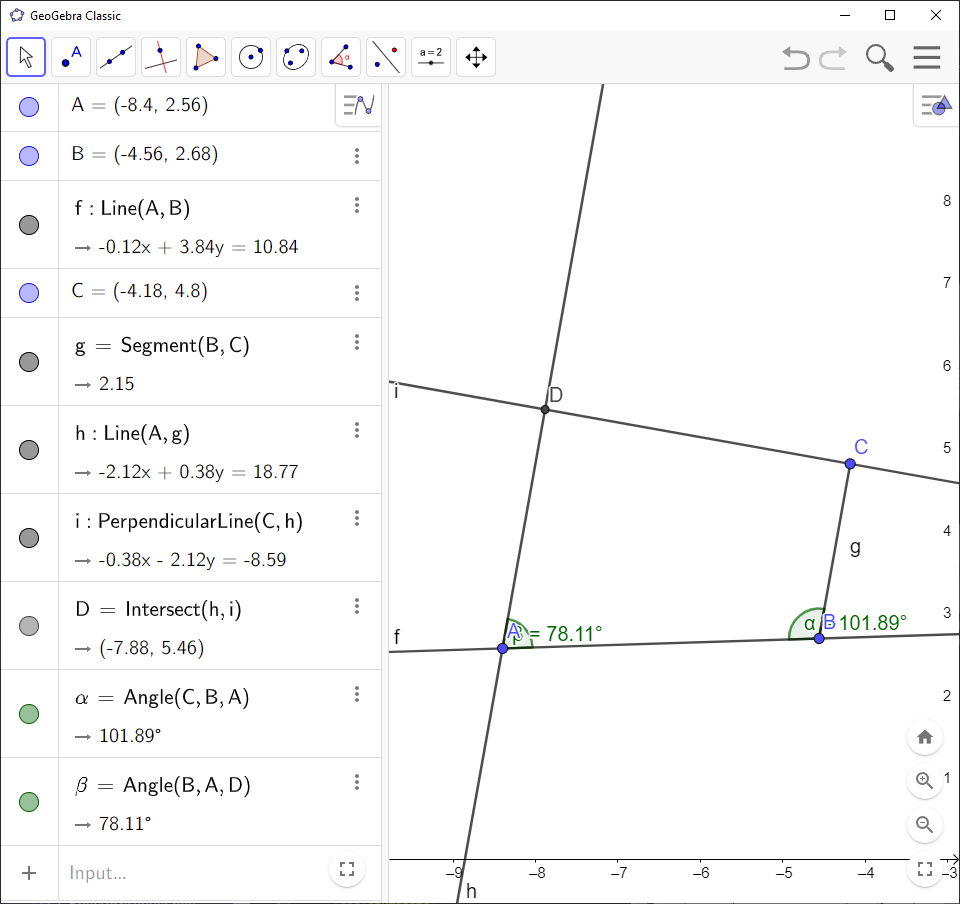Math Topics

# How to Construct Supplementary Angles in GeoGebra

You can easily create and display supplementary angles using `GeoGebra`.

`GeoGebra` Instruction 1

1.
Open `Graphics View` under`View` in`Menu`.
2.
Select `Line`and draw a line in `Graphics View`. You should also get two points on your line named $A$ and $B$.
3.
Select `Point on Object`and draw a point that is between $A$ and $B$. This point will get the name $C$.
4.
Select `Point`and draw a point outside of the line from the previous step. This point will get the name $D$.
5.
Select `Angle`and click

$A$, $C$ and $D$,

in that order. Repeat for the points

$D$, $C$ and $B$.

6.
Select `Move`and drag point $D$ around. You’ll see that the sum of the two angles you drew is always $180$°.

`GeoGebra` Instruction 2

1.
Open `Graphics View` under`View` in`Menu`.
2.
Select `Line`. Click two points in the plane in `Graphics View`, but be sure to click the leftmost point first. You should get a line with two points named $A$ and $B$, where $A$ is the leftmost point of the two.
3.
Select `Point`and draw a point above the line from the previous step. This point will be given the name $C$.
4.
Select `Segment`and click $B$ and $C$.
5.
Select `Parallel line`and click point $A$, then the line segment you drew in the previous step.
6.
Select `Perpendicular Line`and click point $C$, then the parallel line you drew in the previous step.
7.
Select `Intersect`and click the lines from Steps 5 and 6. This point should get the name $D$.
8.
Select `Angle`and click the points

$C$, $B$, and $A$,

in that order. After the angle is drawn, click the points

$B$, $A$, and $D$,

in that order.

9.
Select `Move`and drag point $C$ around while making sure it’s always above the line. You’ll see that the sum of the two angles you drew is always $180$°.